Vers version FRANCAISETweet to @schemath

Fermat's little  theorem (condensed):

In mathematics, the Fermat's little theorem is a result of the modular arithmetic, which can also demonstrate itself with the tools of the elementary arithmetic.

It expresses itself as follows. If 'a' is a not divisible integer by p such as p is a prime number, then a p-1 -1 is a multiple of p. The corollary of this theorem is that, for every 'a' interger and 'p' prime number, then ap -1  is a multiple of p.

He owes his name to Pierre de Fermat (on 1601 - 1665) who expresses it the first time on October 18th, 1640.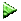I not agree factorized definition :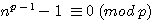:   We can read that, but , if n is divisible by p ,is not true now : example : 10(5-1) -1 is not divisible by 5 what mean?   It's mean that if I divide n by p the rest of this division is 0 example: the rest of 12 / 4 = 0 , it will be noted :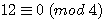# the Fermat's poly polygonals "key" formula

 If we use the Pierre de Fermat's  poly-polygonals formula :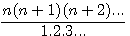and we develop the numerator, then we have a formula like this: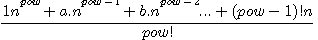(pow mean powered by) Notation n! = Factorielle n instead of 1x2x3x4... n , we write n!  1x2x3x4 = 4! = à 24

Example for=Fermat has proved all his theories (little ,and last theorem) for all power of n , so it is absolutely excluded that Fermat have built them by EACH indices on n. example for 7! indices are 1,21,175,735,1634,1764,720 (see before) . But we can , with a small trick, found the sum off all of them.

How to find the sum of all indices ?

If,we shift n by 1 (n=1) then we getif n=1 thenIf we develop n(n+1)(n+2)...(n+p) , the sum of all indices is equal of the factorial of denominator ,  example for 7! , 1+21+175+735+1634+1764+720 = 5040

Development of numerator:

if p numbers of factors of n(n+1)(n+2).. then

 .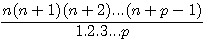=The FIRST exponent = numbers of factors (as Pascal's board),  1pThe FIRST indice is equal to 1The LAST  indice = factorial -1 of numbers of factors.

Exemple : nombre de facteurs = 7<=>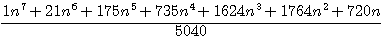The FIRST exponent = numbers of factors : 1n7The FIRST indice is equal to 1 1+21+175+735+1624+1764+720=5040The LAST  indice = factorial -1 of numbers of factors.1+21+175+735+1624+1764+720=5040 , 1 x 2 x 3 x 4 x 5 x 6 =720

Shifting n by 1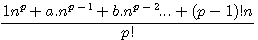if n = 1For example for 7 factorsif n = 1 then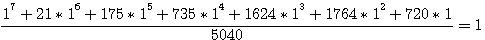,

1+21+175+735+1624+1764+720=5040 = 7!

# Demonstration of Fermat's small theorem

Primaries numbers and factotials

If a number is primary , understand divisible only by himself or 1 , imply that only that the last factor of his factorial is divisible by this number , by example , 7 is primary so 7! = 1 x 2 x 3 x 4 x 5 x 6 x 7 only the last factor , 7 , is divisible by  7 , and NOT the others 2,3,4,5,6 (we get a NOT INTEGER one , we get a decimal number or real )

We deduct also that if a number is primary his factorial LESS ONE is not divisible by this number 2 x 3 x 4 x 5 x 6 is not divisible by 7.

Terms of adding indices and primaries:

If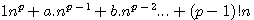from the development of n(n+1)(n+2).... whose p ,numbers of factors, is primary and where we  remplace n by 1

[1+ (a+b+c)+ [(p-1)! ] is divisible by p equal also :   + [a+b+c]+ [(p-1)!]

Concatenation (grouping) in 2 terms

We regroup all the terms of 1+...+ (p-1)! ....  in 2 terms :

 p! = 1 + (a+b+c) = (p-1) x (p-1)! + (p-1)! exemple : 5! = 120 4(1x2x3x4) + 1x2x3x4

We concatenate  1 + a + b+ c ...and we add the last term  (p-1)!

Example : n(n+1)(n+2)(n+3)(n+4) , if n=1 then we get 1(1+1)(1+2)(1+3)(1+4) ; sum of all indices  5! = 120 ,  last indice = 4! =24 then 120 = 96+24

note that if we factorise the twice terms by 24 then 120 = 24(4+1)

120=4x24 + 1x 24 , first =  4x4x3x2x1 , last = 4x3x2x1

That still true for promary numbers of factors or not

##### 5040

Nota:development of n(n+1)(n+2)(n+3)(n+4) = 1n5+10n4+35n3+50n2+24n

Concatenation  in 3 terms , first / intermediate / last

P! is a sum of p terms which the first equal to 1 and the last one  = (p-1)! , We add all intermediate terms in a second term =

p!-1-(p-1)! = p!-(1+(p-1)!)  so 120 = 1 + [120-(1+24)] + 24 = 1 + 95 + 24

The second term is divisible by p (as show bellow) , so we can discard it..

If p is primary , then (p-1)! is not divisible by p , 1 is not divisible by p , 1+ [p!-(1+(p-1)!)] + (p-1)! is divisible by p

<=>

If  + [p!-(1+(p-1)!] + [(p-1)!] ,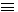0 (mod p) , 1+(p-1)! is divisible par p .

More details

 p! =  ( p=5 => 1x2x3x4x5=120)0 (mod p) 1st + 2nd terms + Last term (p-1)(p-1)!  (5-1)(5-1)(5-2)(5-3)(5-4) = 4x4x3x2x1 = 96 4x4x3x2x1 not divisible by 5 + (p-1)! 4x3x2x1 = 24 4x3x2x1 not divisible by 5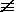0 (mod p) =0 (mod p) 1st  = 1 + + 2nd terms + Last term 1 + p! - 1 - (p-1)! = p! - (1+(p-1)!) 120-1-24= 120 - (25) = 95 + + (p-1)! 1 + p! - 1+(p-1)! + + (p-1)!0 (mod p)0 (mod p) = le premier + dernier terme0 (mod p) p!0 (mod p) => , +[1+(p-1)!]+[(p-1)!] = [1+(p-1)!] + [1+(p-1)!]0 (mod p) , Those 2 terms are equals so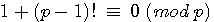Conclusion:   In Fermat's poly-polygonals formula , n(n+1)(n+2)...(n+(p-1)) , if p numbers of factors and if we shift n by 1 then : If p is primary , 1 + 1*2*3...(p-1) is divisible by p n0 (mod p ) <=> the rest of n/p = 0  n0 (mod p ) <=> the rest of n/p is not =0    You can see  this item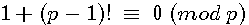For example p=7 then 1+1*2*3*4*5*6 = 721 divisible by 7

Replace now 1 by n = get the Fermat's small theorem

If n(n+1)(n+2)...(n+p-1) = [1np]+[anp-1+bnp-2]+[(p-1)!n] then

 If 1+(p-1)!0 (mod p) then n[1+(p-1)!]0 (mod p) n+n(p-1)!0 (mod p) et aussi : n+n(p-1)!0 (mod n)

In the last term of  1n5+10n4+35n3+50n2+24n , 24n + n = 25n is divisible by 5 , so the first - n is divisible by 5 , it means that if we adding n to the last term for make it divisible we have to subtract n to the fisrt  ( np -n)

 si n+n(p-1)!0 (mod p) alors np - n0 (mod p)

# Prime  number's guess in corollary of Fermat's small theoremn is prime  if(or)

#### Shoud redable as following : if the reminder of  2n-2 / n is 0 <=> if the reminder of 2n-1-1 /n est 0

Ensuing of Fermat's small theorem,if a=2; if remainder (= modulo 1) of a n-a/a  = 0 then n is prime number (if not n is not prime)

It's only a guess because it's necessary to be able to demonstrate it (*),2 n Exceeds the capacities of calculations and calculators...

(I'm writing modulo 1 because it's the computer function , should be)

It's very important to see that in this guess only 2n put numbers into 2 well separed groups , primes and non primes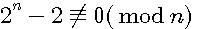(*) Professor Henry Cohen of the university of Bordeaux notes " We can conclude nothing if 2n-1 1 is divisible by n, but it is rather likely in this case that n is prime (Number's history Tallandier Edition 2007)

This conjecture is not true for (n< 1000) 341,561,645 !!!:

# Matrice showing this guess (a=2)

Fermat small theorem don's separate prime and not prime as well as wish.:

Samples :

a=3 n=6 , 6 is not prime , the 36-3/3 reminder should be <> 0

nb: 3 as  2 is prime...

 n 36 36-3 726/6 Rem 6 729 726 121 0

pour a=5 , n=10

 n 510 510 -5 510 -5/5 Rem 10 9765625 9765620 976562 0

It's seems to be the same for all n/a = 2 ...

For a= 4 it's worse...

 n 4n 4n-4 4n-4/4 r 2 16 12 6 0 3 64 60 20 0 4 256 252 63 0 5 1024 1020 204 0 6 4096 4092 682 0 7 16384 16380 2340 0

Small appended notes:

If we want to know if a number is divisible by other one of head you should:

If it's even divide it by 2

If it's odd substract it : "lui ôter une mesure"as would have written it Fermat....)

Ensue of binary algorithm

.

 n 2n 2n  2 2n  2 / n mod 1 2 0 0 0 2 4 2 1 0 3 8 6 2 0 4 16 14 3 1/2 1/2 5 32 30 6 0 6 64 62 10 1/3 1/3 7 128 126 18 0 8 256 254 31 3/4 3/4 9 512 510 56 2/3 2/3 10 1024 1022 102 1/5 1/5 11 2048 2046 186 0 12 4096 4094 341 1/6 1/6 13 8192 8190 630 0 14 16384 16382 1170 1/7 1/7 15 32768 32766 2184 2/5 2/5 16 65536 65534 4095 7/8 7/8 17 131072 131070 7710 0 18 262144 262142 14563 4/9 4/9 19 524288 524286 27594 0 20 1048576 1048574 52428 5/7 5/7 21 2097152 2097150 99864 2/7 2/7 22 4194304 4194302 190650 1/9 1/9 23 8388608 8388606 364722 0 24 16777216 16777214 699050 4/7 4/7 25 33554432 33554430 1342177 1/5 1/5 26 67108864 67108862 2581110 1/9 1/9 27 134217728 134217726 4971026 8/9 8/9 28 268435456 268435454 9586980 1/2 1/2 29 536870912 536870910 18512790 0 30 1073741824 1073741822 35791394 1/9 1/9 31 2147483648 2147483646 69273666 0 32 4294967296 4294967294 134217727 8/9 8/9 33 8589934592 8589934590 260301048 1/6 1/6 34 17179869184 17179869182 505290270 1/9 1/9 35 34359738368 34359738366 981706810 4/9 4/9 36 68719476736 68719476734 1908874353 5/7 5/7 37 137438953472 137438953470 3714566310 0

If mod = 0 then n is prime ... if not n is not

# Important notice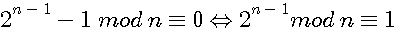by add one on two sides

Is 221 dividable by 7?

221-7=214

214/2=107

107-7=100

50/2=27

27-7=20

20/2=10

10/2=5

221 n'est pas divisible par 7

(31*7 reste 4)

Is 91 dividable by 7?

91-7=84

84/2=42

42/2=21

21-7=14

14-7=7

.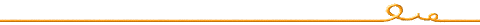Patrick Stoltz le 18/10/2009

pstoltz@shemath.com